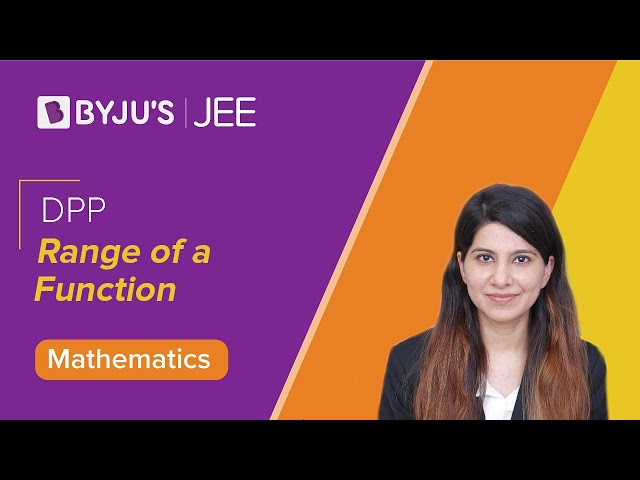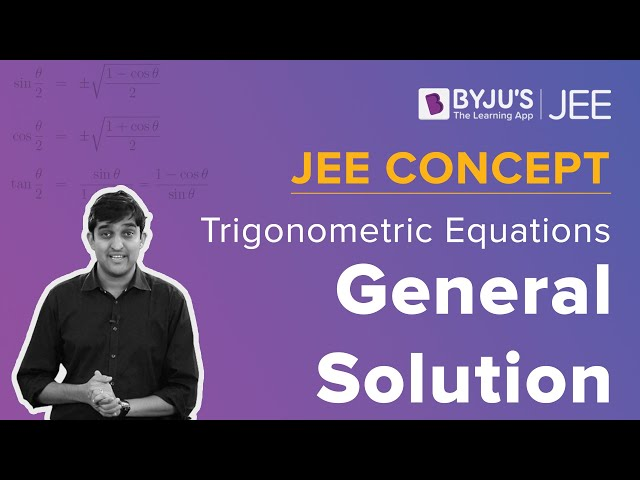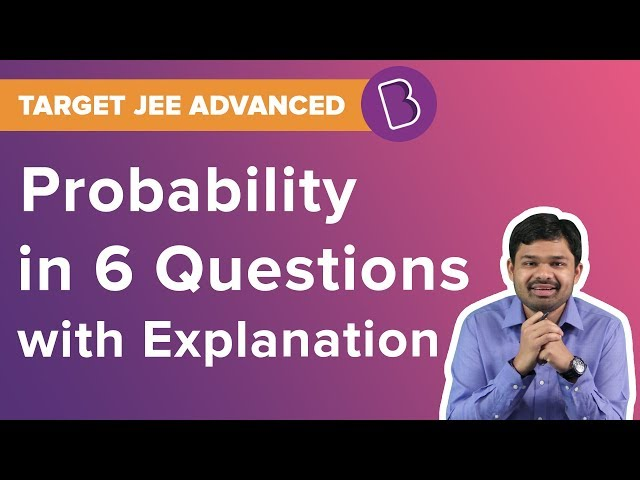Win up to 100% scholarship on Aakash BYJU'S JEE/NEET courses with ABNAT Win up to 100% scholarship on Aakash BYJU'S JEE/NEET courses with ABNAT

# JEE Advanced 2019 Maths Paper 1

Aspirants preparing for JEE Advanced exam can refer to previous year question papers with answers provided by BYJU’S. Referring to last year papers help aspirants for the kind of challenges that the actual exam might throw up and help them in evaluation of performance and preparation level. JEE Advanced Question Paper 2019 Maths Paper 1 solutions are given below. Students can download to practise offline.
Paper 1 – Maths
Question 1: Let M =
$$\begin{array}{l}\begin{bmatrix} sin^4 \theta & -1-sin^2 \theta\\ 1 + cos^2 \theta & cos^4 \theta \end{bmatrix} = \alpha I + \beta M^{-1},\end{array}$$
where α = α(θ) and β = β(θ) are real number, and I is the 2×2 identity matrix. If α* is the minimum of the set {α(θ) : θ Є [0, 2π]} and β* is the minimum of the set {β(θ) : θ Є [0, 2π]} then the value of α* + β* is

a) -37/16
b) -29/16
c) -31/16
d) -17/16

Let M =

$$\begin{array}{l}\begin{bmatrix} sin^4 \theta & -1-sin^2 \theta\\ 1 + cos^2 \theta & cos^4 \theta \end{bmatrix} = \alpha I + \beta M^{-1}\end{array}$$

M2 = αM + βI

a11 = sin θ – 1 – sin2 θ – cos2 θ – cos2 θ sin2 θ = β + α sin4 θ sin8 θ – 2 – cos2 θsin2 θ

= β + α sin4 θ

a21 = sin4 θ + cos2 θ sin4 θ + cos4 θ + cos6 θ = α (1 + cos2 θ)

(1 + cos2 θ) α = sin4 θ (1 + cos2 θ) + cos4 θ (1 + cos2 θ)

α = sin4 θ + cos4 θ = 1 – 2 sin2 θ cos2 θ = 1 – ((sin2 θ)/2)

αmin = ½

β = sin8 θ – 2 – cos2 θsin2 θ – sin8 θ – cos4 θsin4 θ

= -2 – (sin2 2θ)/4 – (sin4 2θ)/16

βmin = -2 – 1/16 [4t2 + t4]

= -2 – 1/16 [t2 + 2]2 + ¼

= -7/4 – 1/16(9) = -37/16

Therefore, α + β = -37/16 + 1/2 = -29/16

Question 2: A line y = mx + 1 intersects the circle (x – 3)2 + (y + 2)2 = 25 at the points P and Q. If the midpoint of the line segment PQ has x-coordinate -3/5, then which one of the following options is correct?
a) 6 ≤ m < 8
b) 2 ≤ m < 4
c) 4 ≤ m < 6
d) -3 ≤ m < -1

y = mx + 1

(x – 3)2 + ((mx +1) + 2)2 = 25

=> x2 (1+ m2 ) + 6(m -1) x – 7 = 0

$$\begin{array}{l}\frac{-3}{5} = \frac{\alpha + \beta}{2} = \frac{-6(m-1)}{2(1+m^2}\end{array}$$

1 + m2 = 5m – 5

Or m = 2, 3

Question 3: Let S be the set of all complex numbers z satisfying |z – 2 + i| ≥ √5. If the complex number z0 is such that 1/|z0 – 1| is the maximum of the set
$$\begin{array}{l}\begin{Bmatrix} \frac{1}{|z-1|}; z \in S \end{Bmatrix}\end{array}$$
then the principle argument of
$$\begin{array}{l}\frac{4-z_0 – \bar{z_0}}{z_0- \bar{z_0} +2i}\end{array}$$

a) π/4
b) -π/2
c) 3π/4
d) π/2

|z – 2 + i| ≥ √5

P is along

$$\begin{array}{l}\bar {AC}\end{array}$$
but at √5 distance from C.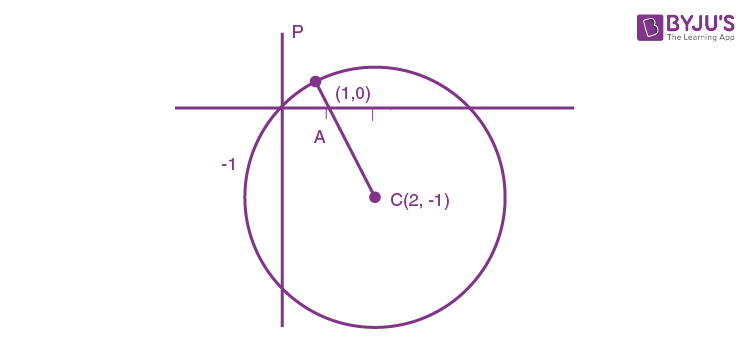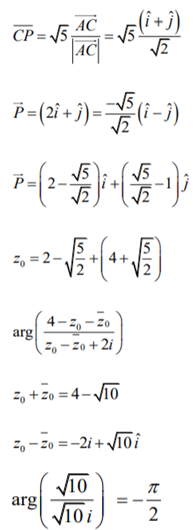Question 4: The area of the region {(x, y) : xy ≤ 8, 1 ≤ y ≤ x2} is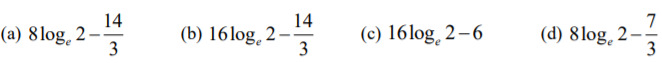{(x, y) : xy ≤ 8, 1 ≤ y ≤ x2}

8/x = x2

$$\begin{array}{l}\int_1^2 (x^2 – 1)dx + \int_2^8(\frac{8}{x} -1)dx\end{array}$$

= 16 loge 2 – 14/3

Question 5: There are three bags B1, B2 and B3. The bag B1 contains 5 red and 5 green balls, B2 contains 3 red and 5 green balls, and B3 contains 5 red and 3 green balls, Bags B1, B2 and B3 have probabilities 3/10, 3/10 and 4/10 respectively of being chosen. A bag is selected at random and a ball is chosen at random from the bag. Then which of the following options is/are correct?
a) Probability that the selected bag is B3 and the chosen ball is green equals 3/10
b) Probability that the chosen ball is green equals 39/80
c) Probability that the chosen ball is green, given that the selected bag is B3, equals 3/8
d) Probability that the selected bag is B3, given that the chosen balls is green, equals 5/13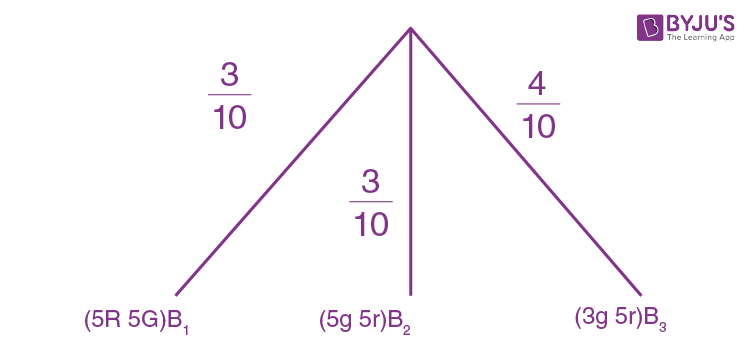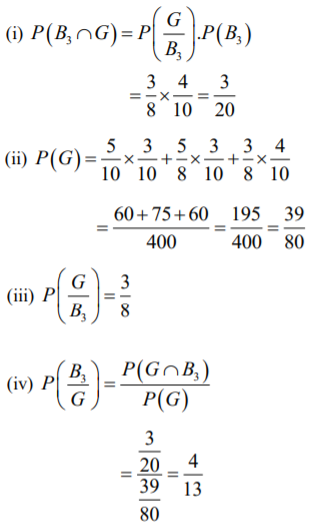Question 6: Define the collections E1, E2, E3 ,….. of ellipses and {R1, R2, R3, …..} of rectangles as follows:

$$\begin{array}{l}E_1 = \frac{x^2}{9}+\frac{y^2}{4} = 1\end{array}$$

R1: Rectangle of largest area, with sides parallel to the axes, inscribed in E1;

En: Ellipse

$$\begin{array}{l}\frac{x^2}{a_n^2}+\frac{y^2}{b_n^2} = 1\end{array}$$
of largest area inscribed in Rn-1, n > 1; Rn: Rectangle of largest area, with sides parallel to the axes, inscribed in En, n > 1.

Then which of the following options is/are correct?

a) The eccentricities of E18 and E19 are NOT equal.
b) The distance of a focus from the centre in E9 is √5/32
c) The length of latus rectum of E9 is 1/6
d)

$$\begin{array}{l}\sum_{n=1}^N\end{array}$$
(area of Rn) < 24, for each positive integer N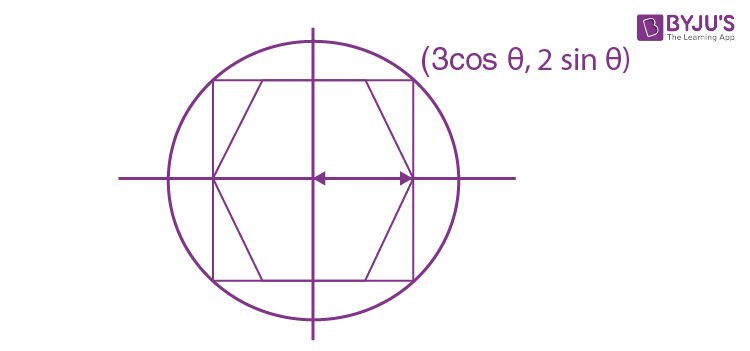A = 6 cosθ . 4 sin θ = 12 sin 2θ -> max

θ = π/4

E2 = a2 = 3 cos(π/4) = 3/√2

b2 = 2 sin θ = √2

$$\begin{array}{l}r = \frac{1}{\sqrt 2}, a_n = \frac{3}{(\sqrt{2})^{n-1}},b_n = \frac{2}{(\sqrt{2})^{n-1}}\end{array}$$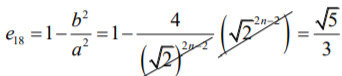So, e18 = 1 – b2/a2 = √5/3

e of all ellipses -> same

Difference of f from conic in equation aqe

$$\begin{array}{l}E_a = \frac{2b_n^2}{a_n} = \frac{3}{(2^8)} \times \frac{\sqrt 5}{3}=\frac{\sqrt 5}{16}\end{array}$$

Length of latus rectum = [2×4]/4(√2) = 1/6

Question 7: Let
$$\begin{array}{l}M = \begin{bmatrix} 0 &1 & a \\ 1& 2 &3 \\ 3 &b &1 \end{bmatrix}\end{array}$$
$$\begin{array}{l}\begin{bmatrix} -1 &1 & -1 \\ 8& -6 &2 \\ -5 &3 &-1 \end{bmatrix}\end{array}$$
where a and b are real numbers. Which of the following options is/are correct?

a) a + b = 3
d) If
$$\begin{array}{l}M \begin{bmatrix} \alpha\\ \beta\\ \gamma \end{bmatrix} = \begin{bmatrix} 1 \\ 2\\ 3 \end{bmatrix}\end{array}$$
, then α – β + γ = 3.

$$\begin{array}{l}M = \begin{bmatrix} 0 &1 & a \\ 1& 2 &3 \\ 3 &b &1 \end{bmatrix}\end{array}$$
$$\begin{array}{l}\begin{bmatrix} -1 &1 & -1 \\ 8& -6 &2 \\ -5 &3 &-1 \end{bmatrix}\end{array}$$

(a)

$$\begin{array}{l}\begin{bmatrix} 2-3b &ab-1 & -1 \\ 8& -6 &2 \\ b-6 &3 &-1 \end{bmatrix}\end{array}$$

b – 6 = -5

=>b = 1

and ab – 1 = 1

or a = 2

Now,

$$\begin{array}{l}M = \begin{bmatrix} 0 &1 & 2 \\ 1& 2 &3 \\ 3 &1 &1 \end{bmatrix}\end{array}$$

|M| = -2

And a + b = 2

(b) |adj M2|= |M2|2 = |M|4 = 16

= 2 M-1 M

= 2 x (-1/2) x M

= -M

(d)

$$\begin{array}{l}M\begin{bmatrix} \alpha \\ \beta \\ \gamma \end{bmatrix} = \begin{bmatrix} 1\\ 2\\ 3 \end{bmatrix}\end{array}$$

This implies,

$$\begin{array}{l}\begin{bmatrix} 0 &1 &2 \\ 1 &2 &3 \\ 3 & 1 & 1 \end{bmatrix}\begin{bmatrix} \alpha \\ \beta \\ \gamma \end{bmatrix} = \begin{bmatrix} 1\\ 2\\ 3 \end{bmatrix}\end{array}$$

β + 2γ = 1

α + 2β + 3γ = 2

3α + β + γ = 1

α – β + γ = 3

Solving all the equations, we have α = 1, β = -1 and γ = 1

Question 8: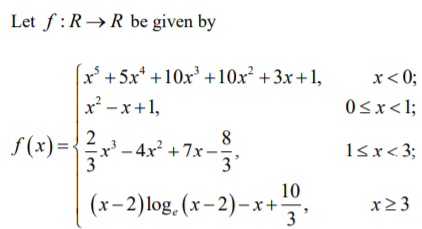Then which of the following options is/are correct?

a) f’ has a local maximum at x = 1
b) f is onto
c) f is increasing on (-∞, 0)
d) f’ is NOT differentiable at x = 1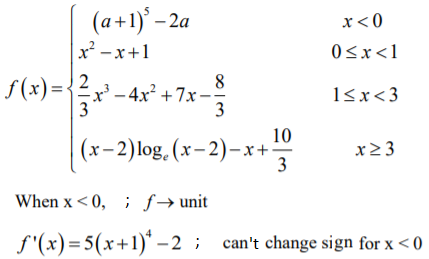Range: -∞, +1

Therefore, Not monotonic.

f(x) = x2 – x + 1

f’(x) = 2x – 1

Max at x = 0 and 1

x ≥ 3

f(3) = 1 log 1 – 3 + 10/3 = 1/3

f(∞) -> ∞

$$\begin{array}{l}f'(x) = \left\{\begin{matrix} 2x-1 & ; 0 \leq x <1\\ 2x^2-8x+7 & ;1 \leq x<3 \end{matrix}\right.\end{array}$$

Loc. Max at x = 1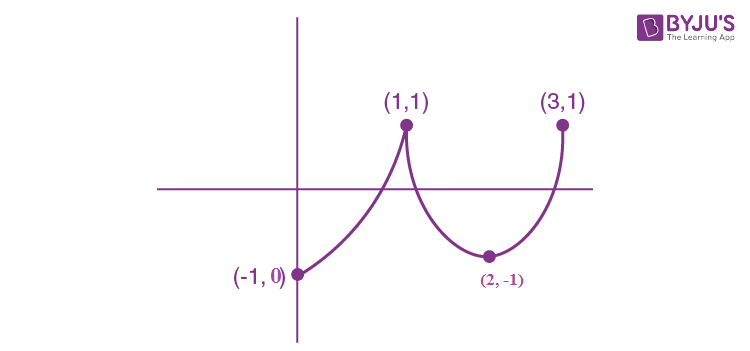Question 9: Let α and β be the roots of x2 – x – 1 = 0, with α > β. For all positive integers n, define

$$\begin{array}{l}a_n = \frac{\alpha^n – \beta^n}{\alpha – \beta}, n \geq 1\end{array}$$

b1 = 1 and bn = an-1 + an+1, n ≥ 2.

Then which of the following options is/are correct?

a)

$$\begin{array}{l}a_1 + a_2 + a_3 +….+a_n = a_{n+2} – 1\end{array}$$
for all n ≥ 1

b)
$$\begin{array}{l}\sum_{n=1}^\infty \frac{a_n}{10^n} = \frac{10}{89}\end{array}$$

c)
$$\begin{array}{l}\sum_{n=1}^\infty \frac{b_n}{10^n} = \frac{8}{89}\end{array}$$

d)
$$\begin{array}{l}b_n = \alpha^n + \beta^n\end{array}$$
for all n ≥ 1.

x2 – x – 1 = 0

α = [1+ √5]/2 and β = [1- √5]/2

$$\begin{array}{l}a_n = \frac{\alpha^n – \beta^n}{\alpha – \beta}\end{array}$$

As, b1 = 1 and bn = an-1 + an+1, n ≥ 2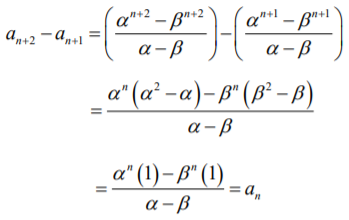a1 + a2 + …+ an =

$$\begin{array}{l}\sum_{n=1}^\infty \frac{a_n}{10^n}\end{array}$$

=> an + an+1 = an+2

=>

$$\begin{array}{l}\frac{\sum (\frac{\alpha}{10})^n – \sum(\frac{\beta}{10})^n}{\alpha – \beta}\end{array}$$
$$\begin{array}{l}\sum_{r=1}^n a_r= a_{n+2} – a_2\end{array}$$

= an+2 – [α2 – β2]/[α -β]

= an+2 – [α + β]

= an+2 – 1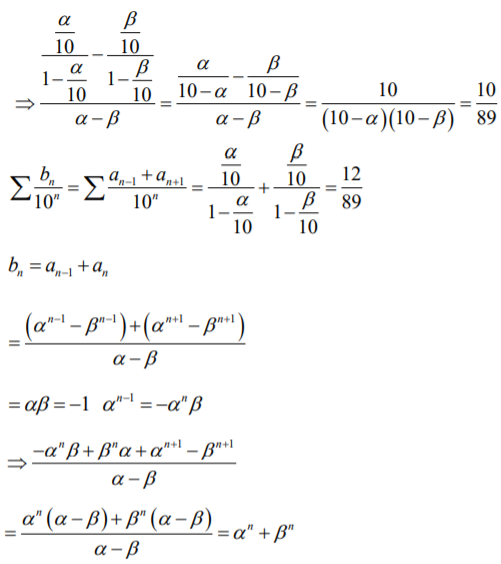Question 10: Let denote a curve y = y(x) which is in the first quadrant and let the point (1, 0) lie on it. Let the tangent to at a point P intersect the y-axis at YP. If PYP has length 1 for each point P on , then which of the following is options is/are correct?
a)
$$\begin{array}{l}y = log_e(\frac{1+\sqrt{1-x^2}}{x}) – \sqrt{1-x^2}\end{array}$$

b) xy’ – √[1-x2] = 0
c)
$$\begin{array}{l}y = log_e(\frac{1+\sqrt{1-x^2}}{x}) + \sqrt{1-x^2}\end{array}$$

d) xy’ + √[1-x2] = 0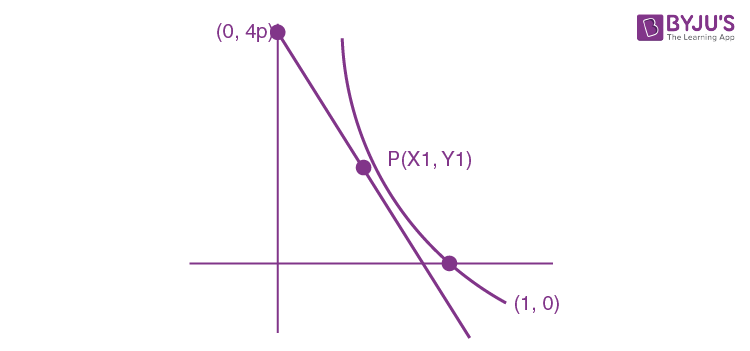y – y1 = m(x – x1)

yp – y1 = -mx1

=> 1 = x12 + m2 x12

1 = x2[1 + (dy/dx)2]

Or (dy/dx)2 = 1/x2 – 1

dy/dx = ± √[1-x2]/x

$$\begin{array}{l}y = \pm \int \frac{\sqrt{1-x^2}}{x} \ dx\end{array}$$

Here x = sinθ, dx = cos θ dθ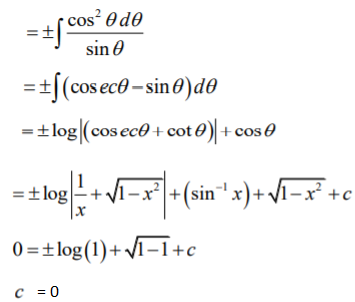=>

$$\begin{array}{l}y = log_e(\frac{1+\sqrt{1-x^2}}{x}) – \sqrt{1-x^2}\end{array}$$

And the correct differential equation will be xy’ + √[1-x2] = 0

Question 11 In a non-right-angle triangle ΔPQR, let p, q, r denote the lengths of the sides opposite to the angles at P, Q, R respectively. The median from R meets the side PQ at S, the perpendicular from P meets the side QR at E, and RS and PE intersect at 0. If p = √3, q = 1, and the radius of the circumcircle of the ΔPQR equals 1, then which of the following options is/are correct?
a) Area of ΔSOE = √3/12
b) Radius of incircle of ΔPQR = √3/2 [2 – √3]
c) Length of RS = √7/2
d) Length of OE = 1/6

(sin P)/√3 = (sin Q)/1 = 1/2R = ½ ; [Given R = 1]

∠P = π/3 or 2π/3

∠Q = π/6 or 5π/6

p>q=> ∠P = ∠Q

If ∠P = π/3 and ∠Q = π/6 => ∠R = π/2

Therefore, ∠P = 2π/3 and ∠Q = ∠R = π/6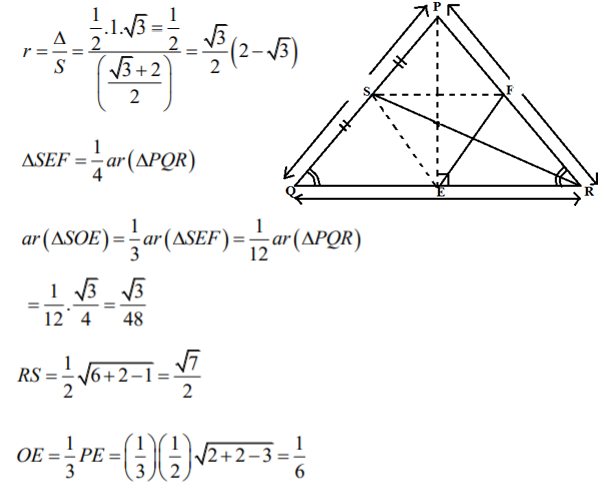Question 12: Let L1 and L2 denotes the lines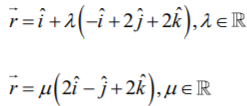respectively. If L3 is a line which is perpendicular to both L1 and L2 and cuts both of them, then which of the following options describe(s) L3?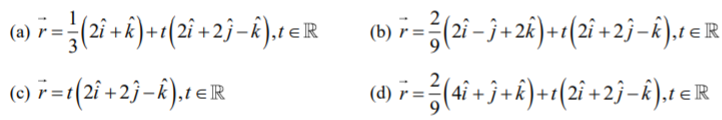$$\begin{array}{l}L_1 : \vec r = \hat i + \lambda(- \hat i + 2 \hat j + 2 \hat k)\end{array}$$
$$\begin{array}{l}L_2 : \vec r = \mu(-2 \hat i – \hat j + 2 \hat k)\end{array}$$

L1 : [x-1]/-1 = [y-0]/2 = [z-0]/2

L2 : x/2 = y/-1 = z/2

L3 : x/a = y/b = z/c

L3 = L1 x L2

Similarly,

$$\begin{array}{l}6 \hat i + 6 \hat j – 3 \hat k\end{array}$$

A on L1 : (-λ + 1, 2λ, 2λ)

B on L2 : (2μ, -μ, 2μ)

AB Δrs : (2μ, + λ – 1 -μ – 2λ, 2μ – 2λ) = (6, 6, -3) or (2, 2, -1)

=>

$$\begin{array}{l}\frac{2 \mu + \lambda – 1}{2} = \frac{- \mu – 2 \lambda}{2} = \frac{2 \mu – 2 \lambda}{-1} = k\end{array}$$

λ = [3k+1]/3 and μ = -4k – 2/3

Now, 2 μ – 2 λ + k = 0

Putting μ and λ values, we have

k = -2/9=> μ = 2/9 and λ = 1/9

So, A: (8/9, 2/9, 2/9) and B : (4/9, -2/9, 4/9)

Mid point is (2/3, 0, 1/3)

For equation of L3;

vector A is the position vector of any point on L3.

Question 13: If
$$\begin{array}{l}I = \frac{2}{\pi} \int_{-\pi/4}^{\pi/4} \frac{dx}{(1 + e^{sin\ x})(2-cos 2x)}\end{array}$$
then 27I2 equals _________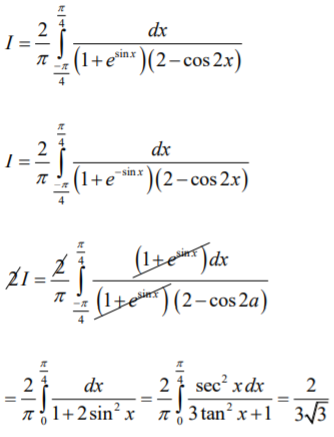27I2 = 4

Question 14: Let the point B be the reflection of the point A(2, 3) with respect to the line 8x – 6y – 23 = 0. Let A and B be circle of radii 2 and 1 with centres A and B respectively. Let T be a common tangent to the circle A and B such that both the circles are on the same side of T. If C is the point of intersection of T and the line passing through A and B, then the length of the line segment AC is ________.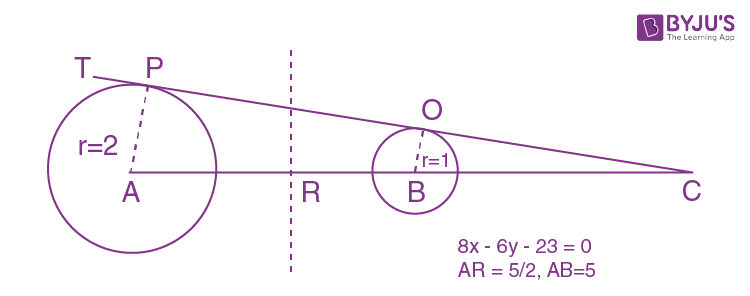ΔAPC and ΔBQC are similar.

BC/AC = 1/2 => 2(AC – AB) = AC

AC = 2AB = 10

Question 15: Let AP (a; d) denote the set of all the terms of an infinite arithmetic progression with first term a and common difference d > 0. If AP(1; 3) ∩ AP (2; 5) ∩ AP (3; 7) = AP (a; d) then a + d equals_________

I: 1, 4, 7, 10, 13, 16 ….. 32

II: 2, 7, 12, 17, 22, 27, 32, 37, 42, 47, 52

III: 3, 10, 17, 24, 31, 38, 45, 52

52 <– a + d –> LCM of 3, 5, 7 = 105

So, a + d = 157

Question 16: Let S be the sample space of all 3 × 3 matrices with entries from the set {0, 1}. Let the events E1 and E2 be given by

E1 = {A ϵ S : det A = 0} and

E2 = {A ϵ S : sum of entries of A is 7}.

If a matrix is chosen at random from S, then the conditional probability P(E1|E2) equals …….

S: 29

$$\begin{array}{l}P(\frac{E_1}{E_2}) = \frac{P(E_1 \cap E_2)}{P(E_2)}\end{array}$$

E2 : Sum of entries 7, we need 7 – 1s and 2 0’s

Total E2 = 9!/7!2! = 36

$$\begin{array}{l}\begin{vmatrix} 1 &1 &1 \\ 1& 1 &1 \\ 1& 0 &0 \end{vmatrix}\end{array}$$

Per |A| to be zero, both zeroes should be in the same row/column.

Therefore, 3 x 3 x 2 = 18 cases

$$\begin{array}{l}P(\frac{E_1}{E_2})\end{array}$$
= 18/36 = ½

Question 17: Three lines are given by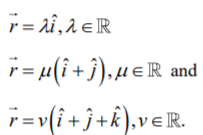Let the lines cut the plane x + y + z 1 at the points A, B and C respectively. If the area of the triangle ABC is Δ then the value of (6Δ)2 equals __________1st line: x = λ, y = 0, z = 0

x + y + z = 1 => λ = 1

A(1, 0, 0)

2nd line: x = μ, y = μ, z = 0

2μ = 1 or μ = ½

B(1/2, ½, 0)

Parallels, C(1/3, 1/3, 1/3)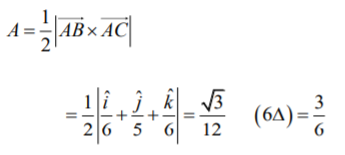Question 18: Let ω ≠ 1 be a cube root of unity. Then the minimum of the set {|a + bω + cω2|2 ; Where, a, b, c distinct non-zero integers }equals ________

| a + bω + cω2|2

= (a + bω + cω2) (a + cω + bω2)

= (a2 + b2 + c2 – ab – bc – ca)

[because, conjugate of (a + bω + cω2) = (a + cω + bω2)]

= > (1/2)[(a-b)2 + (b-c)2 + (c-a)2] = ½(1+1+4) = 3

[For minimum value a = 1, b = 2 and c = 3]

## Video Lessons – Paper 1 Maths